Science Journal of Applied Mathematics and Statistics
Volume 3, Issue 4, August 2015, Pages: 199-203

The Application of ARIMA Model in 2014 Shanghai Composite Stock Price Index

Renhao Jin*, Sha Wang, Fang Yan, Jie Zhu

School of Information, Beijing Wuzi University, Beijing, China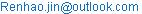(Renhao Jin)

Renhao Jin, Sha Wang, Fang Yan, Jie Zhu. The Application of ARIMA Model in 2014 Shanghai Composite Stock Price Index. Science Journal of Applied Mathematics and Statistics. Vol. 3, No. 4, 2015, pp. 199-203. doi: 10.11648/j.sjams.20150304.16

Abstract: In order to study the changes of Shanghai Composite Stock Price Index (SCSPI) and predict the trend of stock market fluctuations, this paper constructed a time-series analysisA non-stationary trend is found, and an ARIMA model is found to sufficiently model the data. A short trend of Shanghai composite stock price index is then predicted using the established model.

Keywords: The Shanghai Composite Stock Price Index (SCSPI), Prediction, ARIMA Model

Contents

1. Introduction

There are more than 3000 stocks in China stock market, and Shanghai Composite Stock Price Index (SCSPI) is a good representatives for all the stocks in China market. SCSPI is the first release of the stock index in China, and it is calculated based on all the stocks in Shanghai stock market. In the financial industry of China, the prediction of Shanghai composite stock price index is always a high-profile topic, which is useful in avoiding the risk of investment interests, and also in reflecting the changes of structure, activities and trends of China macro-economic. If investors can accurately predict the stock market trend, the invest risk can be reduced and the benefits can be maximized. Thus, scientific and reasonable forecast of stock index really vital to financial practices.

A lot of methods have been used for SCSPI, including autoregressive model, autoregressive moving average model, autoregressive conditional heteroscedasticity model, autoregressive integrated moving average model (ARIMA) and so on. In the balance of predict and explanation, ARIMA is a wildly used model. In this paper, the SCSPI data of 2014 is firstly examined and then ARIMA model is used to fitted the data.

Stock price forecasting are most concerned about the stock opening price, closing price, the highest price, the lowest price and volume. In technical analysis, the highest and lowest price represents the comprehensive fighting among multi outer forces. The transacted volume represents the market activity and popularity, and the closing price is on behalf of the balance from the multi contest, which can be seen as the opening price of the next trading day. According to the technical analysis theory and the basic hypothesis, a trading day closing price is not only associated with the previous trading day closing price, the highest price, the lowest price and volume, but also with the historical trading day’s closing price, the highest price, low price and volume. In view of the important role in the analysis of stock market closing price, the SCSPI data used in this paper is based on closing prices of the stocks, in the time span of January 2, 2014 to December 31, 2014, with 234 observations. The Data is obtained from the financial part of Sina.com. A short list of the data can be seen in Table 1. All computations are done by using SAS software (SAS® 9.2, SAS Institute Inc., Cary, N.C.).

2. ARIMA Modeling

2.1. ARIMA Model

In statistics and econometrics, and in particular in time series analysis, an autoregressive integrated moving average (ARIMA) model is a generalization of an autoregressive moving average (ARMA) model. These models are fitted to time series data either to better understand the data or to predict future points in the series (forecasting). They are applied in some cases where data show evidence of non-stationarity, where an initial differencing step (corresponding to the "integrated" part of the model) can be applied to reduce the non-stationarity. Non-seasonal ARIMA models are generally denoted ARIMA (p, d, q) where parameters p, d, and q are non-negative integers, p is the order of the Autoregressive model, d is the degree of differencing, and q is the order of the Moving-average model. Seasonal ARIMA models are usually denoted ARIMA (p, d, q) (P, D,Q) , where m refers to the number of periods in each season, and the uppercase P, D, Q refer to the autoregressive, differencing, and moving average terms for the seasonal part of the ARIMA model. ARIMA models form an important part of the Box-Jenkins approach to time-series modelling.

When two out of the three terms are zeros, the model may be referred to based on the non-zero parameter, dropping "AR", "I" or "MA" from the acronym describing the model. For example, ARIMA (1,0,0) is AR(1), ARIMA(0,1,0) is I(1), and ARIMA(0,0,1) is MA(1).

Table 1. A short list of the SCSPI data in 2014, which contains transaction date, open price, maximum price, close price, minimum price, volume and transaction amount.

 Date Open Price Max Price Close price Min price Volume Transaction Amount (China Yuan) 2014/1/2 2112.126 2113.11 2109.387 2101.016 6848548800 61921353728 2014/1/3 2101.542 2102.167 2083.136 2075.899 8449724000 72372232192 2014/1/6 2078.684 2078.684 2045.709 2034.006 8958760800 72895397888 2014/1/7 2034.224 2052.279 2047.317 2029.246 6340293600 54638641152 2014/1/8 2047.256 2062.952 2044.34 2037.11 7164736000 62941429760 2014/1/9 2041.773 2057.196 2027.622 2026.446 7594188000 67605663744 2014/1/10 2023.535 2029.297 2013.298 2008.007 7561612000 61044551680 2014/1/13 2014.978 2027.181 2009.564 2000.404 6654477600 55616749568 2014/1/14 2007.156 2027.428 2026.842 2001.135 7036661600 56687624192 2014/1/15 2024.228 2027.409 2023.348 2010.204 6743622400 57740357632 2014/1/16 2022.538 2034.707 2023.701 2014.407 7275572000 62829760512 2014/1/17 2017.522 2017.868 2004.949 2001.33 6730572000 57039564800 2014/1/20 2001.894 2005.938 1991.253 1984.824 5627124800 48298782720 2014/1/21 1992.015 2014.152 2008.313 1992.015 5984491200 52017917952 2014/1/22 2009.969 2052.339 2051.749 2008.93 9889285600 84126662656 2014/1/23 2048.331 2052.528 2042.18 2039.052 8421058400 76083322880 2014/1/24 2037.667 2060.986 2054.392 2034.453 9294792000 82346180608 2014/1/27 2044.272 2044.846 2033.3 2029.626 8881542400 81914216448 2014/1/28 2036.402 2047.129 2038.513 2026.987 7252904000 65751605248 2014/1/29 2042.176 2051.583 2049.914 2039.771 7386546400 67694125056 2014/1/30 2045.931 2045.931 2033.083 2031.466 6261518400 58264498176

2.2. Building Stationary Sequence

A timing diagram is firstly plot using all the SCSPI data of 2014 based on closing stocks price. As shown in Figure 1, a clear increasing trend can be found from the diagram, which is corresponding the increasing China economics. The SCSPI changes from around 2100 points in the beginning to around 3250 points in the end of year 2014. The increasing trend breaks the hypotheses of weaker stationary. In many application cases, the weaker stationary is used instead of strongly stationary.

A weaker form of stationarity commonly employed in time series is known as second-order stationarity, which only require that 1st moment and auto-covariance do not vary with respect to time. So, for a continuous-time random process  x(t),  it has the following properties: the mean function E{x(t)} must be constant and the covariance function depends only on the difference between t1 and t2 and only needs to be indexed by one variable rather than two variables.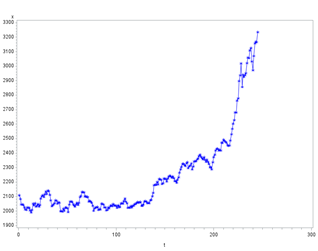Figure 1. The timing diagram plot of SCSPI data of 2014 based on closing stocks price.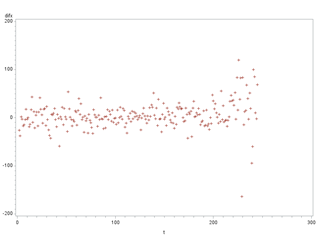Figure 2. The timing diagram plot of the First order differencing data on SCSPI data of 2014 based on closing stocks price.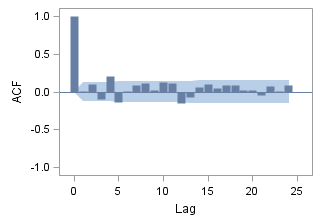Figure 3. The Autocorrelogram on the first order differencing data of original SCSPI data.

Following ARIMA model procedure, a First order differencing is computed for the data, and then a timing diagram of the differencing data is computed and shown in Figure 2. The differencing data shows a stationary pattern, and an Autocorrelogram (Figure 3) is also done on the differencing data, which displays a short-term autocorrelation and confirms the stationary of the differencing data. To make an accurate inference of the data, autocorrelation check for white noise is also done on the differencing data. As shown in Table 2, the white noise hypotheses is rejected on lag 6, 12, 18 and 24 with very small p-values. All these results shows that an ARMA model can be fitted to the first order differencing data.

2.3. ARIMA Modeling

The basic idea of ARIMA model is to view the data sequence as formed by a Stochastic Process on time. Once the model has been identified, the model can be used to predict the future value from the past and present value of the time series. Modern statistical methods and econometric models have been able to help companies predict the future in a certain way.

Firstly, the scatter plots of time series, self-correlation function and partial auto correlation function plot are used to test its variance, trend and seasonal variation, stability of sequence recognition. For general applications, the time series of economic events are not stationary series. The next step is to do some data manipulation on the non-stationary sequence. If the data series is non-stationary, and there is a certain growth or decline, the data difference is need to be proceed. If the heteroskedasticity is in the data, the technical data processing is required. After the data processing, the correlation function value and partial correlation function values should be not significantly different from zero.

Table 2. Autocorrelation check for white noise on the differencing data at lag 6, 12, 18 and 24.

 Autocorrelation Check for White Noise Lag Chi-Square DF P-Value Autocorrelations 6 20.85 6 0.0019 0.012 0.101 -0.099 0.210 -0.138 0.007 12 39.10 12 0.0001 0.089 0.116 0.018 0.123 0.113 -0.147 18 48.73 18 0.0001 -0.076 0.056 0.1 0.048 0.085 0.090 24 52.98 24 0.0006 0.019 0.024 -0.041 0.067 0.014 0.091

According to the identification rules on time series, the corresponding model can be established. If a partial correlation function of a stationary sequence is truncated, and self-correlation function is tailed, it can be concluded the sequences for AR model; if partial correlation function of a stationary sequence is tailed, and the self- correlation function is truncated, it can be determined that the MA model can be fitted for the sequence. If the partial correlation function of a stationary sequence and the auto-correlation function are trailed, then the ARMA model is suitable for the sequence.

Based on the results from section 2.2, an ARIMA model can be fitted to the original SCSPI data of 2014, and the parameters in ARIMA (p, 1, q) need to be determined then. From the Figure 3 of the Autocorrelogram, it is safe to determine that q is no more than 3, while as shown in Figure 4, the partial autocorrelation is also no more than 3. This means that it is enough to choose the model in the set of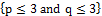.

At the significant level of 0.05, no model in the forms of ARIMA (p, 1, 0) can be found which makes the coefficients of AR part are significantly different from 0. The same result can also be found in the models with the forms of ARIMA (0, 1, q). In the models in ARIMA (p, 1, q), two models ( ARIMA (1,1,1) and ARIMA (2,1,1) ) are found with all coefficients are significantly different from 0. The model ARIMA (1,1, 1) is chosen as it contains fewer coefficients than ARIMA (2,1,1), although the AIC of the former model are larger than the later one but only with the amount of 0.439, 0.02% the AIC amount of ARIMA (2,1,1).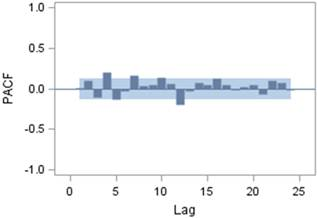Figure 4. The partial Autocorrelogram on the first order differencing data of original SCSPI data.

The final model is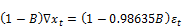,

which can also be written as.

Using the built model, five steps prediction can be done and compared with the actual value, which are listed in Table 3 and Figure 5. As shown in Table 3, the forecast value is around 3255 and with a relative small standard error, which is also reflected in the 95% confidence limits.

Table 3. The next 5 steps prediction of actual SCSPI value based on the final model. The predicted value are shown with the standard error and 95% confidence limits.

 Forecasts for variable x(t) Obs Forecast Std Error 95% Confidence Limits 246 3245.2351 27.3885 3191.5545 3298.9156 247 3255.7931 38.9985 3179.3575 3332.2287 248 3266.3511 48.0887 3172.0990 3360.6032 249 3276.9091 55.9048 3167.3378 3386.4804 250 3287.4671 62.9255 3164.1354 3410.7987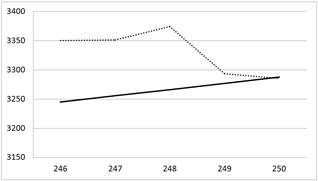Figure 5. The dash line is draw by the actual SCSPI value, while the solid line is from the predicted values.

Although the predicted values is a little different from actual value, the increasing trend is agreed in the 3 steps, which is enough for financial practice. The fluctuation of SCSPI is can be caused by many factors, such as China financial police, international financial events and polices. The fluctuations of stocks are non-rational, and it is influenced by many factors. No model can include all these factors. The most frequent reason which makes the stock suddenly appeared on big sell-off is the country and the global financial policy and current affairs. For example, in January 19, 2015, a lot of stocks in Shanghai stock markets are plummeted, and all financial stocks, such as brokerage stocks and bank stocks are plummeted. The Shanghai Composite Index closing price decreased from 3376.495 points down to 3116.351 points, by 260.144 points, and fell 7.70%. The main reasons is that on last weekend China Securities Regulatory Commission announced the two financial inspection and punishing results: CITIC Securities, Haitong Securities, and Guotai Junan Securities are not allowed to open new credit accounts for three months, and a number of brokers have been criticized. In addition, at the same time Swiss central bank unexpectedly announced that CHF cancelled against the euro exchange rate cap, which make CHF rise and the euro exchange rate hit a new low. By the effect of this unexpected news and weaker economic data, the United States stock indexes decreased in the next five days. The resulting turmoil in the international financial markets are also affected to China stocks markets in some extent.

3. Conclusions

This paper does a study on 2014 Shanghai Composite Stock Price Index (SCSPI). In the process of model building, the original SCSPI data is found to be un-stationary, but the first order differencing data of original SCSPI data is stationary. By comparing with several models, ARIMA (1, 1, 1) is chosen as the final model and it succeeds in predicting three steps trends of SCSPI. Considering the fluctuations of SCSPI, this model can be applied in finance practices. The fluctuations of stocks are non-rational, and it is influenced by many factors. No model can include all these factors. Although the predicted values from the suggested model is a little different from actual value, the increasing trend is agreed in the 3 steps, which is enough for financial practice. That is because in the financial practice it is very perfect to make an investment to assure a coming profit.

Acknowledgements

This paper is funded by the project of National Natural Science Fund, Logistics distribution of artificial order picking random process model analysis and research (Project number: 71371033); and funded by intelligent logistics system Beijing Key Laboratory (No.BZ0211); and funded by scientific-research bases---Science & Technology Innovation Platform---Modern logistics information and control technology research (Project number: PXM2015_014214_000001); University Cultivation Fund Project of 2014-Research on Congestion Model and algorithm of picking system in distribution center (0541502703).

References

1. Apergis, N., Mervar, A., & Payne, J. E. (2015). Forecasting disaggregated tourist arrivals in Croatia: evidence from seasonal univariate time series models. Tourism Economics.
2. Akaike, H. (1973), "Information Theory and an Extension of the Maximum Likelihood Principle," in B.N. Petrov and F.Csaki, ed. 2nd International Symposium on Information Theory, 267-281. Akademia Kiado: Budapest.
3. Box, G.E.P., Jenkins, G.M., and Reinsel, G.C.(1994), Time Series Analysis: Forecasting and Control, 3rd edition, Prentice Hall: Englewood Cliffs, New Jersey.
4. Box, G.E.P., and Pierce, D. (1970), "Distribution of Residual Autocorrelations in Auto-regressive-Intergrated Moving Average Time Series Models," Journal of the American statistical Association, 65, 1509-1526.
5. Cox, D. R., & Wermuth, N. (1991). A simple approximation for bivariate and trivariate normal integrals. International Statistical Review/Revue Internationale de Statistique, 59(2), 263-269.
6. Franke, J., Härdle, W. K., & Hafner, C. M. (2015). ARIMA Time Series Models. In Statistics of Financial Markets (pp. 237-261). Springer Berlin Heidelberg.
7. Tsay, R.S., and Tiao, G.C. (1984), "Consistent Estimates of Auto-regressive Parameters and Extended Sample Auto-correlation Function for Stationary and Non-stationary ARMA models," Journal of American Statistical Association, 79, 84-96.
8. SAS Institute Inc, (2008). SAS/STAT® 9.2 User’s Guide: The ARIMA Procedure (Book Excerpt). NC: SAS Institute Inc, Cary.
9. Bollerslev T. Generalized autoregressive conditional heteroskedasticity [J]. Journal of Econometrics, 1986, 31 (3): 309-317.
10. Bollerslev T. Modelling the Coherence in Short-Run Nominal Exchange Rates: A Multivariate Generalized ARCH Mode [J]. Review of Economics and Statistics,1990, 72: 499-503.
11. Bollerslev T., Engle R.F., Wooldridge M.J. A capital Asset Pricing Model with time-varying covariances [J]. Journal of Political Economy, 1988, 96: 119-130.
12. Engle R.F. Autoregressive conditional heteroskedasticity with estimates of the variance of United Kingdom inflation [J]. Econometric, 1982, 50 (4): 989-1004.
13. Engle R.F., Kroner F.K. Multivariate Simultaneous Generalized ARCH [J].Econometric Theory, 1995, 11:135-149.
14. Engle R.F., Lilien D.M., Robins R.P. Estimating time-varying risk Premia in the term structure: The ARCH-M model [J]. Econometrica, 1987, 55: 395-406.
15. Engle Robert F. Dynamic Conditional Correlation: A Simple Class of Multivariate GARCH Models [J]. Journal of Business and Economic Statistics, 2002, 20 (3):341-347.
16. Glosten L. R., Jagannathan R. and Runkle D. E. On the relation between expected value and the volatility of the nominal excess return on stocks [J]. The Journal of Finance, 1993, 48 (5): 1779-1801.
17. Nelsen R.B. An introduction to Copulas [M]. New York: Springer-Verlag, 1999.
18. Nelson B. Conditional heteroscedasticity in asset returns: a new approach [J]. Econometrica, 1991, 59: 349-360.
19. Nelson D.B. ARCH models as diffusion approximations [J]. Journal of Econometrics, 1990, 45: 9-28.
20. Wang, W. C., Chau, K. W., Xu, D. M., & Chen, X. Y. (2015). Improving Forecasting Accuracy of Annual Runoff Time Series Using ARIMA Based on EEMD Decomposition. Water Resources Management, 29(8), 2655-2675.
21. Zakoian J.M. Threshold heteroskedastic models [J]. Journal of Economic Dynamics and Control, 1990, 18: 937-945.

 Contents 1. 2. 2.1. 2.2. 2.3. 3.
Article ToolsAbstractPDF(283K)